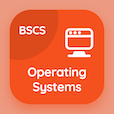Computer Science Online Courses

Digital Logic Design MCQs

Digital Logic Design MCQ PDF - Topics

# Decimal Codes MCQ Quiz Online

Learn Decimal Codes Multiple Choice Questions (MCQ), Decimal Codes quiz answers PDF to study digital logic design online course for digital logic design classes. Binary Systems Multiple Choice Questions and Answers (MCQs), Decimal Codes quiz questions for BSc computer science. "Decimal Codes MCQ" PDF Book: binary code, subtraction with complement, definition of binary logic, logic gates in digital logic design test prep for software engineering online courses.

"Decimal number 5 in Binary Coded Decimal (BCD) 8421 coding is equal to" MCQ PDF: decimal codes with choices 101, 1001, 1010, and 1100 for BSc computer science. Study decimal codes quiz questions for merit scholarship test and certificate programs for software engineering online courses.

## MCQs on Decimal Codes Quiz

MCQ: Decimal number 5 in Binary Coded Decimal (BCD) 8421 coding is equal to

101
1001
1010
1100

MCQ: Decimal number 9 in BCD 8421 coding is equal to

1101
1001
1100
1110

MCQ: The Bi-quinary code is the seven-bit code with

Error correction properties
Error detection properties
Error transmission properties
Parity transmission properties

### More Topics from Digital Logic Design Course